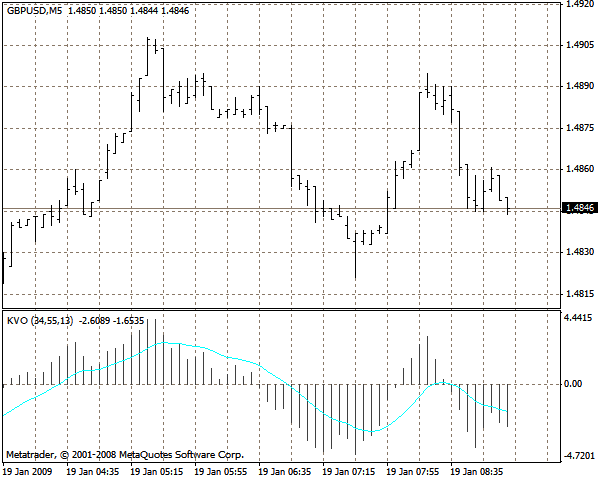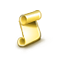Interesting script?
So post a link to it -
let others appraise it

You liked the script? Try it in the MetaTrader 5 terminal# Klinger Oscillator KVO - indicator for MetaTrader 4

Views:
25818
Rating:
Published:
2016.07.01 10:51
Updated:
2016.11.22 07:32

This oscillator based on turnovers has been developed by Stephen Klinger.

The oscillator is calculated in seven steps:

1. The average typical price is calculated - (H+L+C)/3
2. If the current typical price is greater than the average typical price of the previous bar, the turnover of the current bar gets a "plus" sign
3. If the current typical price is less than the average typical price of the previous bar, the turnover of the current bar gets a "minus" sign
4. A 34-period EMA of the turnover obtained in steps 2 or 3 is calculated
5. A 55-period EMA of the turnover obtained in steps 2 or 3 is calculated
6. The 34-period EMA is subtracted from the 55-period EMA; the result is plotted on the chart as a histogram
7. The EMA of the difference obtained in step 6 is calculated and plotted on the chartTranslated from Russian by MetaQuotes Software Corp.
Original code: https://www.mql5.com/ru/code/8677BETTER_YZGraphOnLine

The script visualizes the deals on the chart in a convenient way.The Cronex T Demarker GF indicator

Variation of the DeMarker indicatorThe Cronex T RSI GF indicator

Popularly demanded modification of the RSI indicatorLocker

It can be used as a standalone EA or as a method of recovery from losses